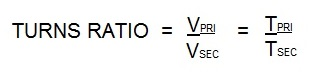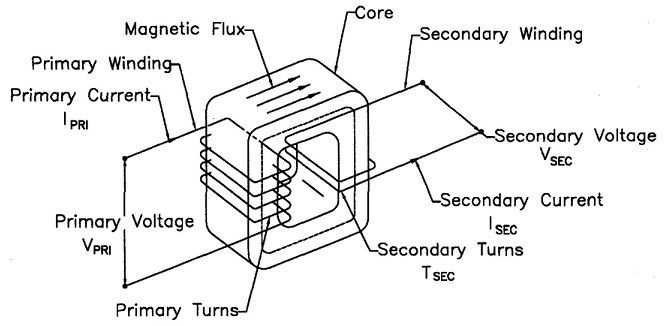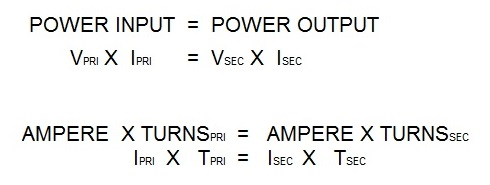# What is the turns ratio for a spot welder?

To answer this question I will demonstrate the calculation of the turns ratio for a AC 16 KVA resistance welding transformer.

The turns ratio for all resistance welding transformers is calculated in the same manner no matter the KVA size. Turns ratio is the ratio of the number of coil turns in the primary vs the secondary.In AC transformers, there are many turns in the primary. The secondary has one turn. If there are 50 turns then the ratio is 50/1. Therefor the primary voltage will drop from 220V and 100 amp input to:

220/50 = 4.4 volts in the secondary. The equations for this are:Vpri = Voltage on the primary                          Tpri = Turns in the primary coil
Vsec= Voltage on the secondary                     Tsec = Turns in the secondary coilPower into the transformer will equal power out of the transformerIsec = Secondary amperage                 Amperage Ipri = Primary amperage

In the secondary using the above formula:
If the Ipri = 100 amperes
If the turns ratio = 50/1
Isec = (Ipri X Tpri)/ Tsec
Isec = 100 x 50/1
Isec = 5000 amperes

There are several additional articles written on turns ratio available in the “HOW TO RESISTANCE WELD” blog.

Reference: RWMA Manual Chapter 19

### Have a Question?

Do you have a question that is not covered in our knowledgebase? Do you have questions regarding the above article? Click here to ask the professor.